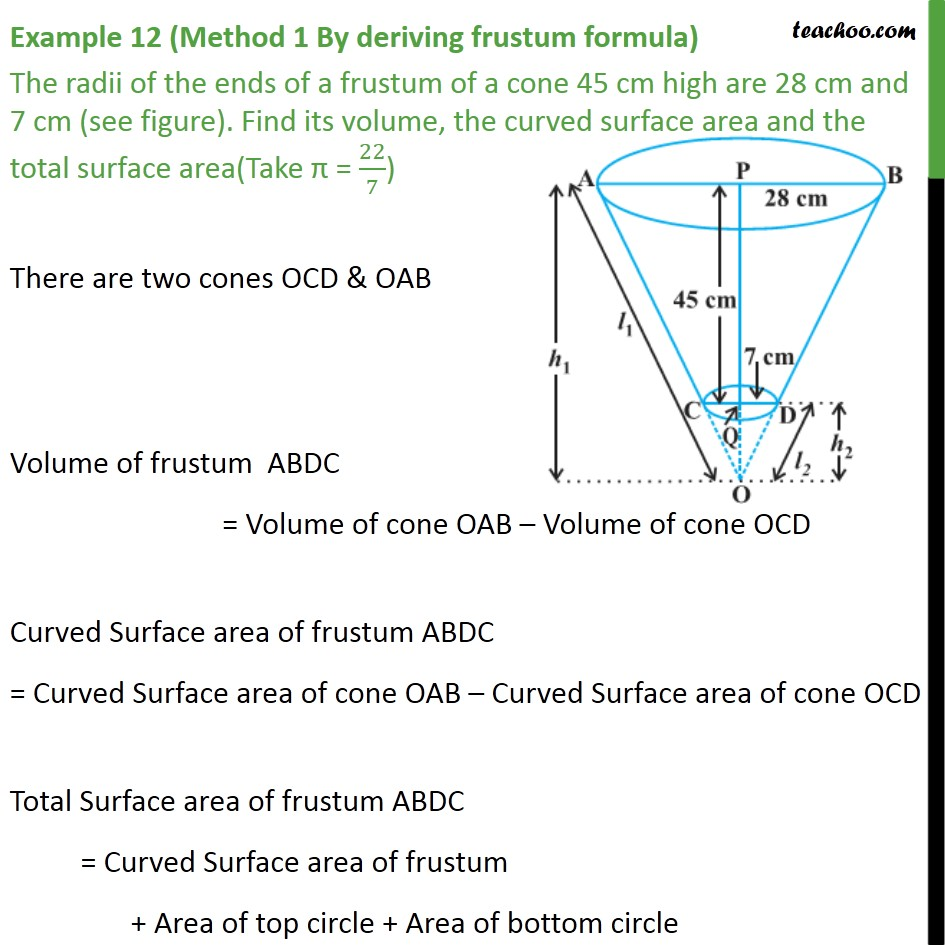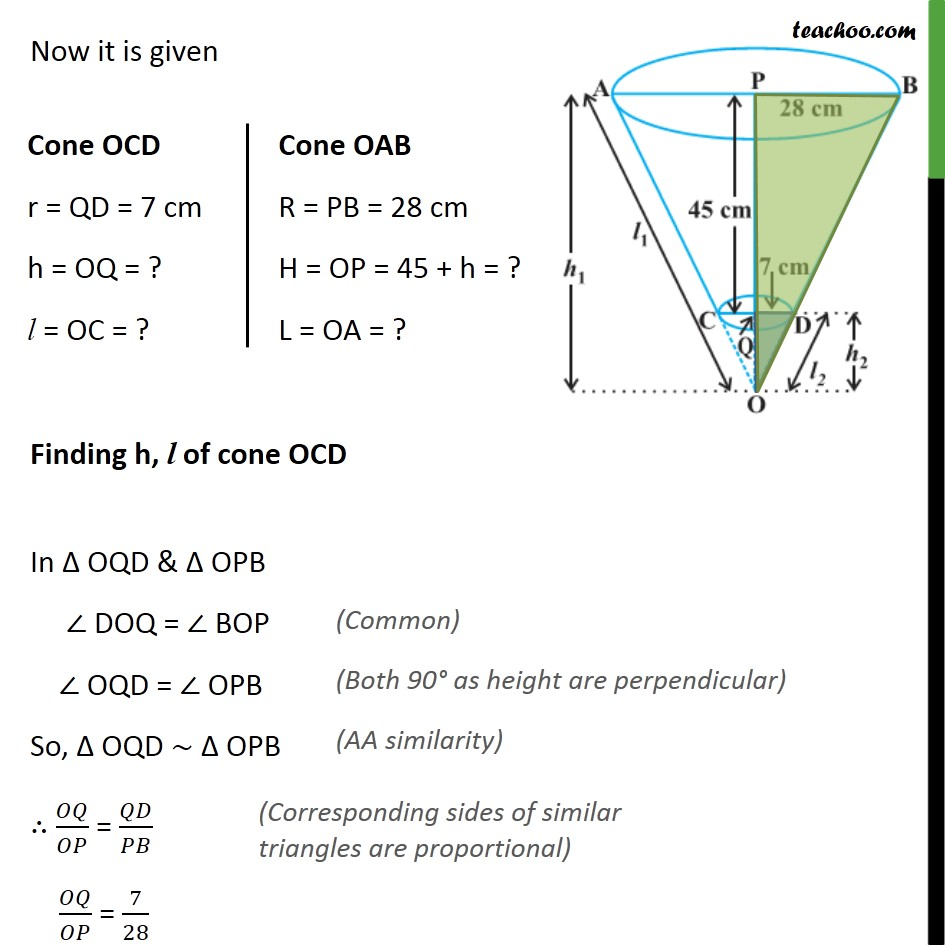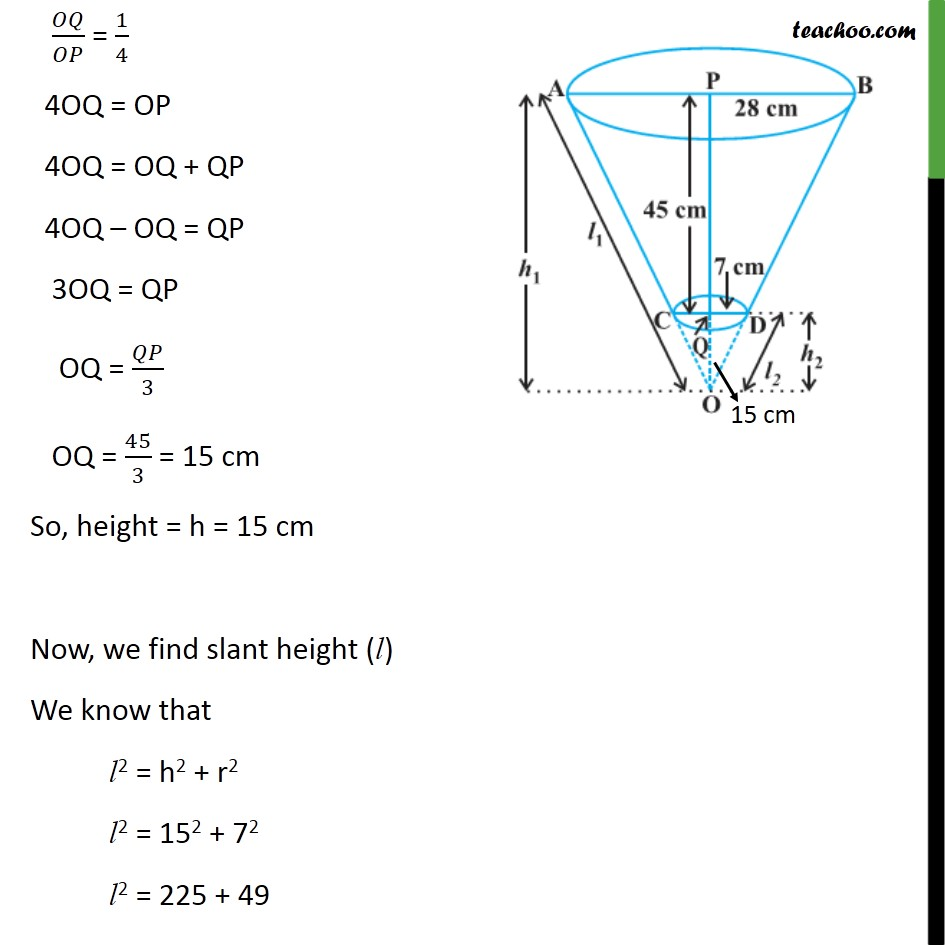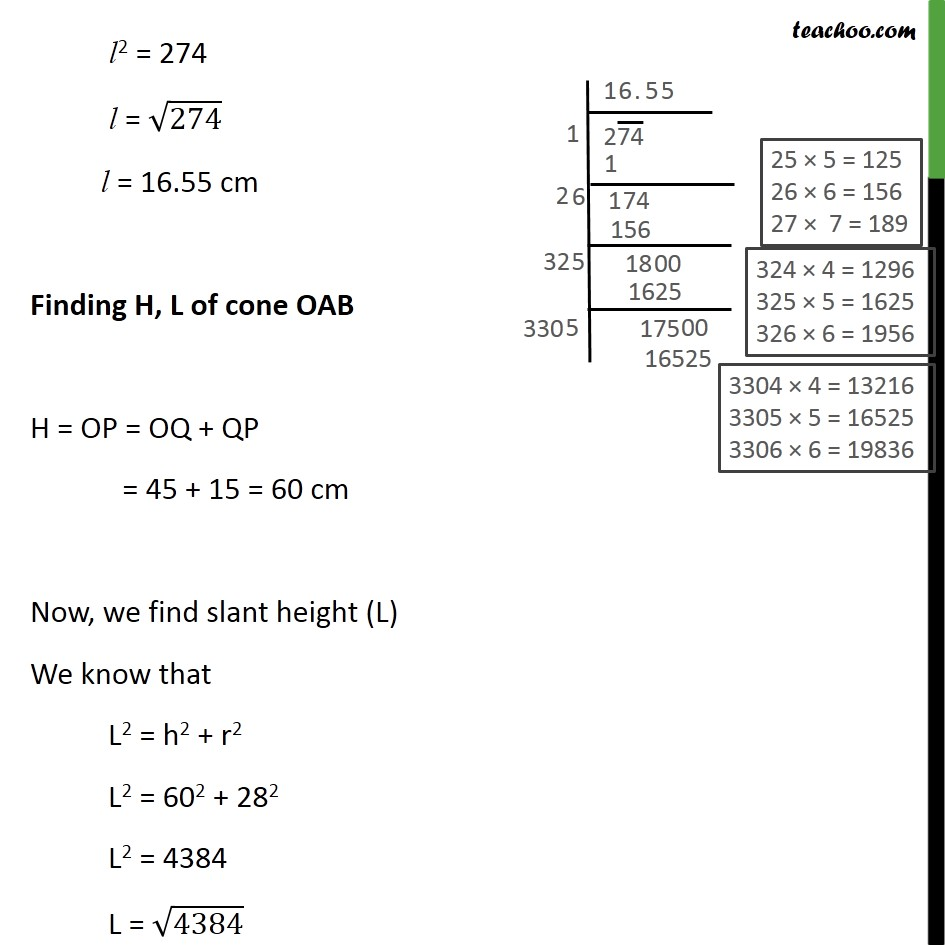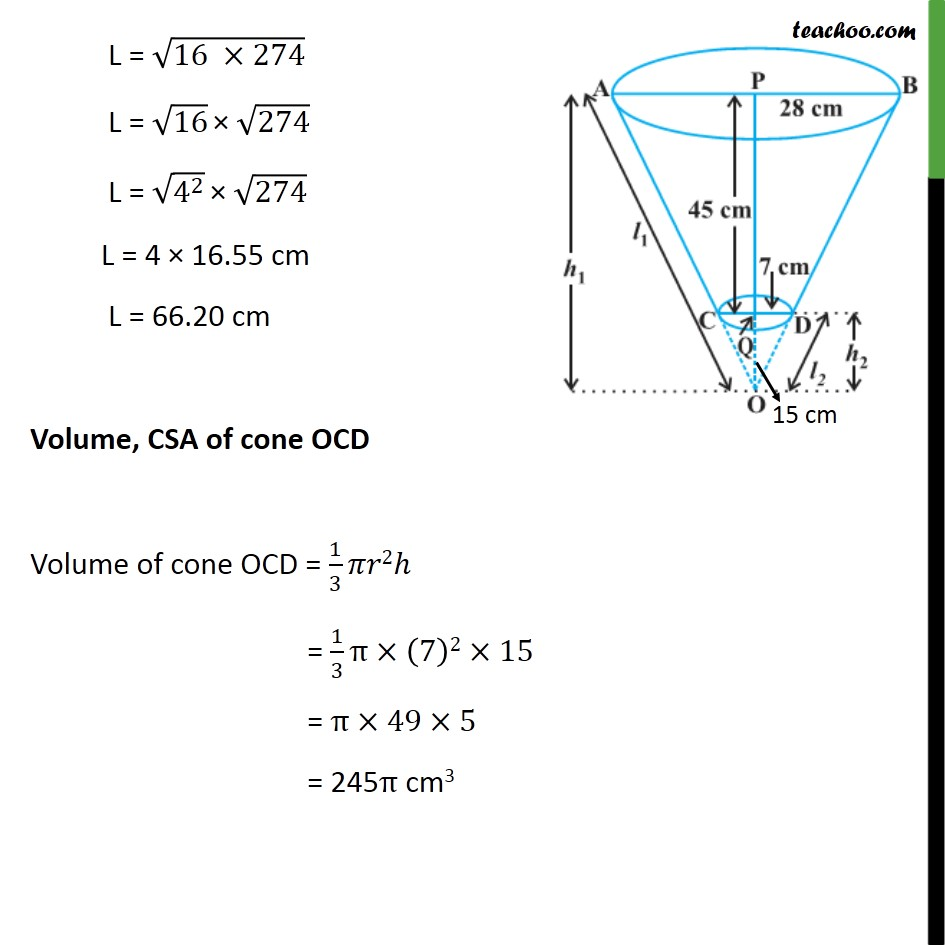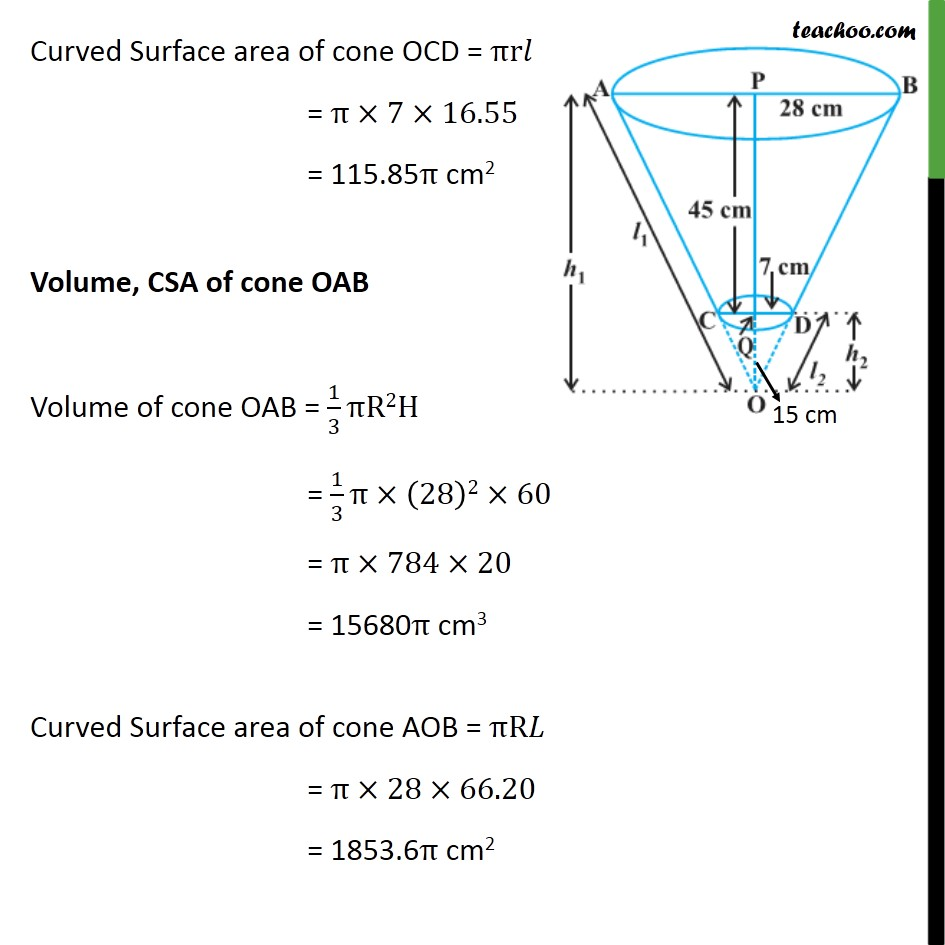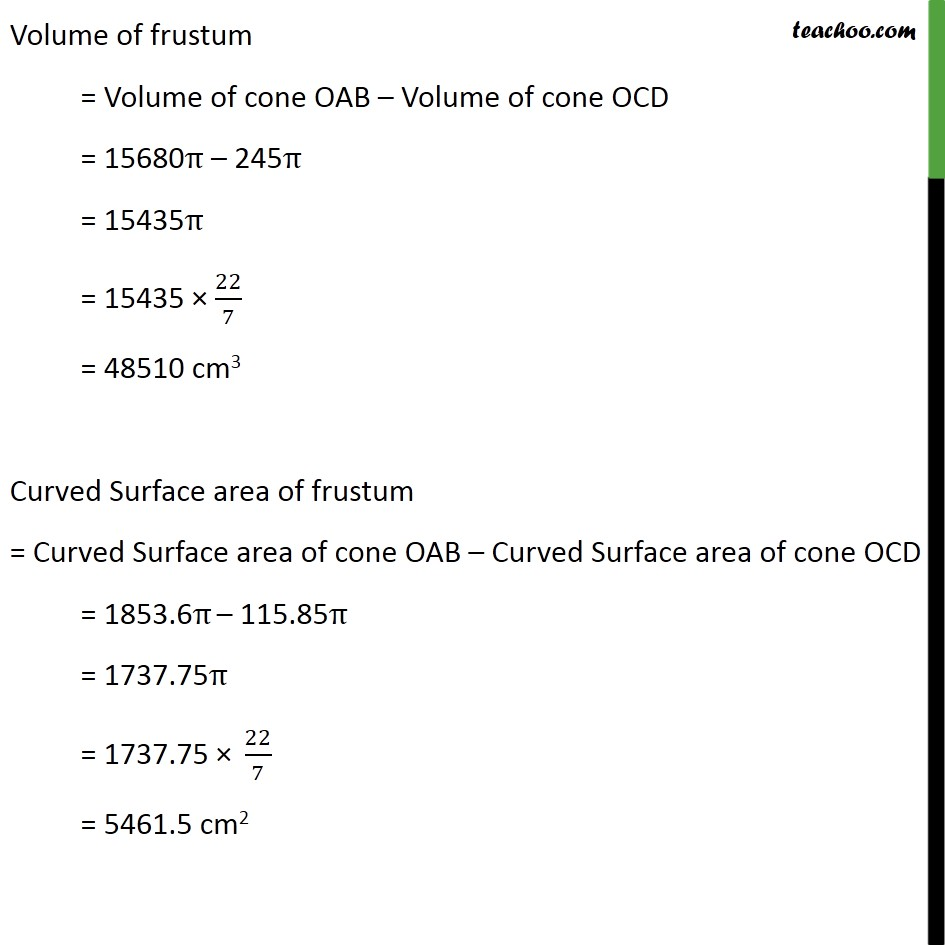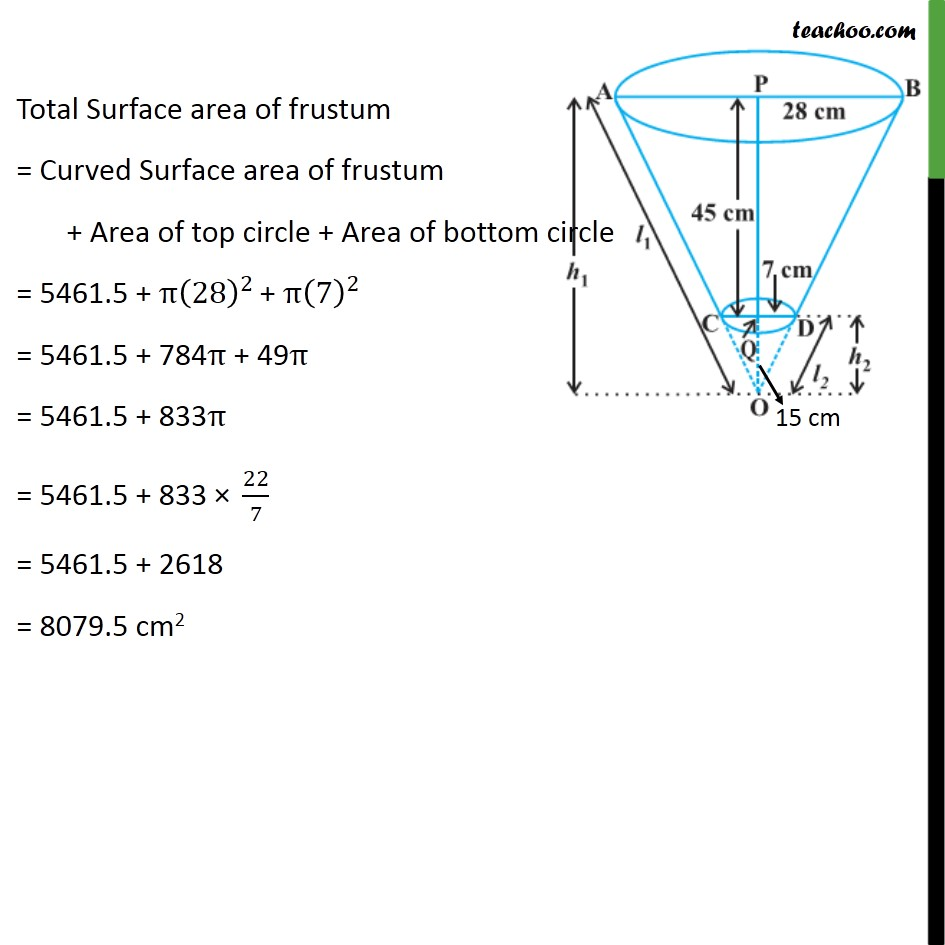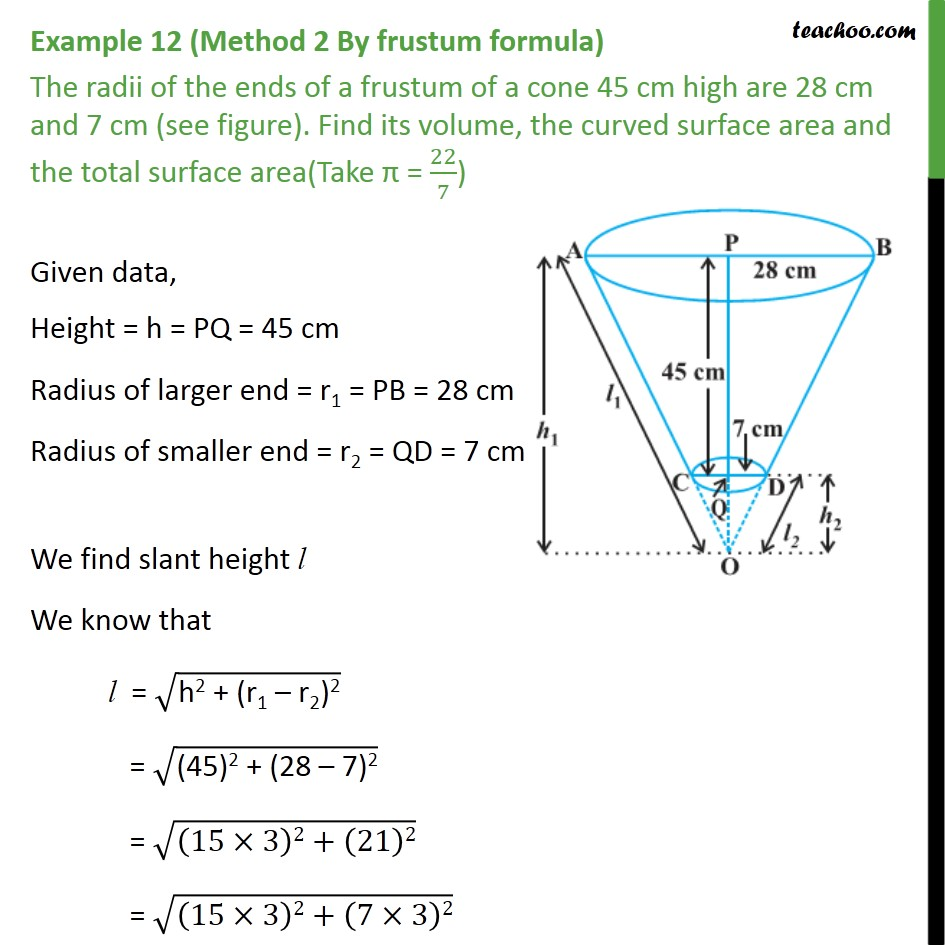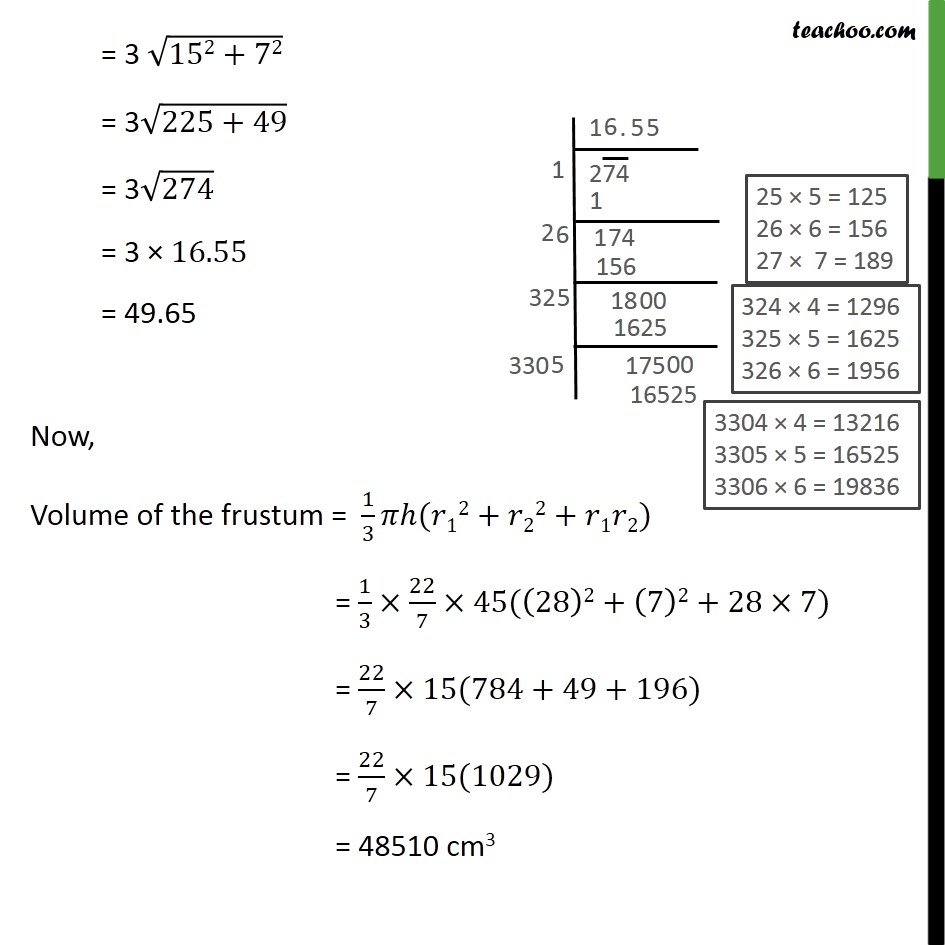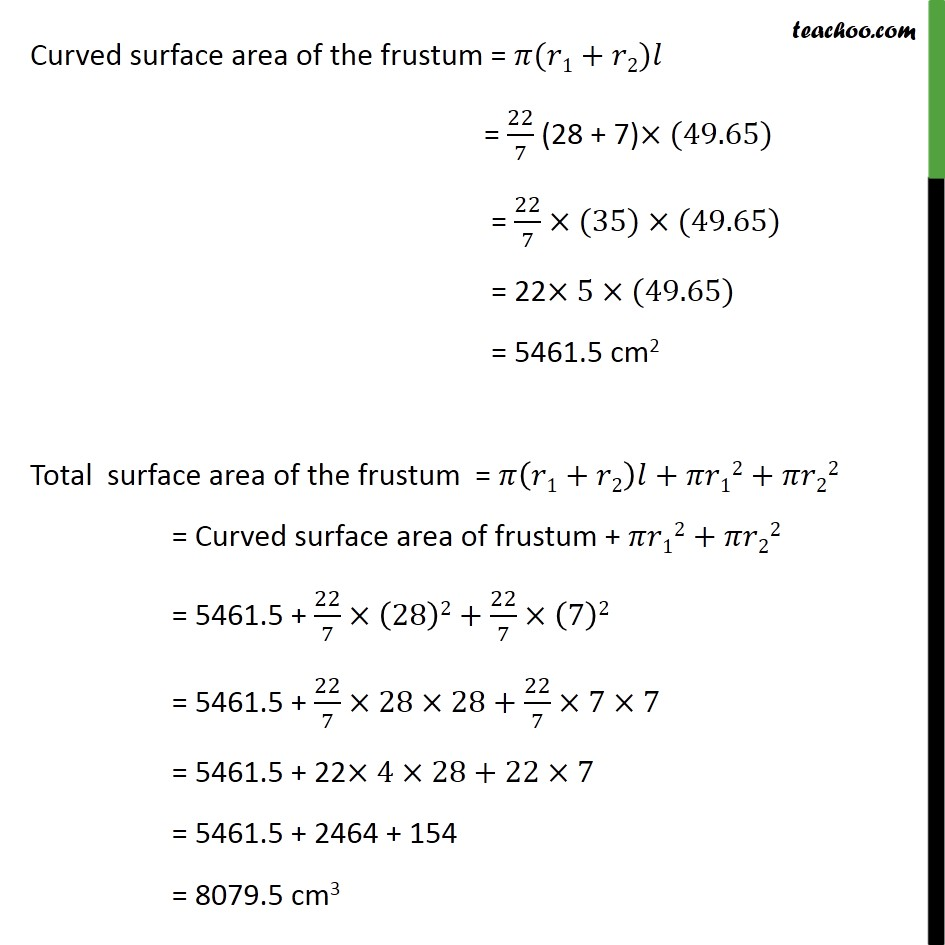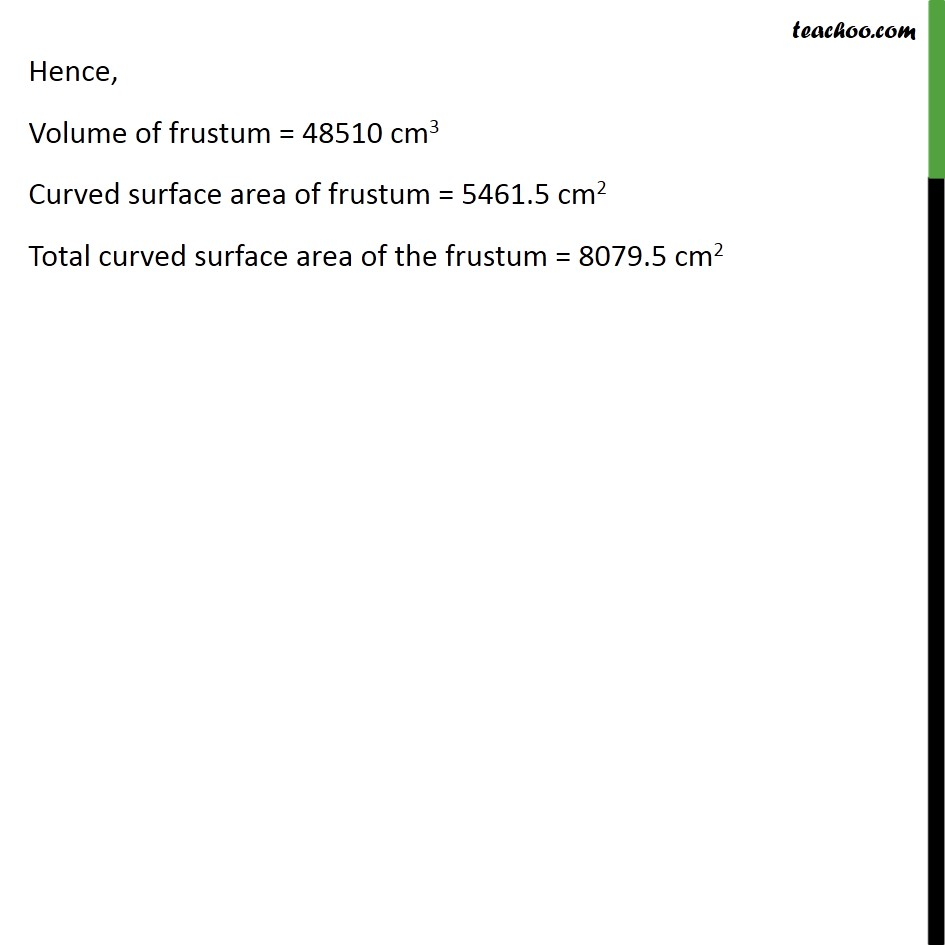1. Class 10
2. Important Questions for Exam - Class 10
3. Chapter 13 Class 10 Surface Areas and Volumes

Transcript

Example 12 (Method 1 By deriving frustum formula) The radii of the ends of a frustum of a cone 45 cm high are 28 cm and 7 cm (see figure). Find its volume, the curved surface area and the total surface area(Take = 22/7) There are two cones OCD & OAB Volume of frustum ABDC = Volume of cone OAB Volume of cone OCD Curved Surface area of frustum ABDC = Curved Surface area of cone OAB Curved Surface area of cone OCD Total Surface area of frustum ABDC = Curved Surface area of frustum + Area of top circle + Area of bottom circle Now it is given Finding h, l of cone OCD In OQD & OPB DOQ = BOP OQD = OPB So, OQD OPB / = / / = 7/28 / = 1/4 4OQ = OP 4OQ = OQ + QP 4OQ OQ = QP 3OQ = QP OQ = /3 OQ = 45/3 = 15 cm So, height = h = 15 cm Now, we find slant height (l) We know that l2 = h2 + r2 l2 = 152 + 72 l2 = 225 + 49 l2 = 274 l = 274 l = 16.55 cm Finding H, L of cone OAB H = OP = OQ + QP = 45 + 15 = 60 cm Now, we find slant height (L) We know that L2 = h2 + r2 L2 = 602 + 282 L2 = 4384 L = 4384 L = (16 274) L = 16 274 L = (4^2 ) 274 L = 4 16.55 cm L = 66.20 cm Volume, CSA of cone OCD Volume of cone OCD = 1/3 2 = 1/3 (7)2 15 = 49 5 = 245 cm3 Curved Surface area of cone OCD = r = 7 16.55 = 115.85 cm2 Volume, CSA of cone OAB Volume of cone OAB = 1/3 R2H = 1/3 (28)2 60 = 784 20 = 15680 cm3 Curved Surface area of cone AOB = R = 28 66.20 = 1853.6 cm2 Volume of frustum = Volume of cone OAB Volume of cone OCD = 15680 245 = 15435 = 15435 22/7 = 48510 cm3 Curved Surface area of frustum = Curved Surface area of cone OAB Curved Surface area of cone OCD = 1853.6 115.85 = 1737.75 = 1737.75 " " 22/7 = 5461.5 cm2 Total Surface area of frustum = Curved Surface area of frustum + Area of top circle + Area of bottom circle = 5461.5 + (28)^2 + (7)^2 = 5461.5 + 784 + 49 = 5461.5 + 833 = 5461.5 + 833 " " 22/7 = 5461.5 + 2618 = 8079.5 cm2 Example 12 (Method 2 By frustum formula) The radii of the ends of a frustum of a cone 45 cm high are 28 cm and 7 cm (see figure). Find its volume, the curved surface area and the total surface area(Take = 22/7) Given data, Height = h = PQ = 45 cm Radius of larger end = r1 = PB = 28 cm Radius of smaller end = r2 = QD = 7 cm We find slant height l We know that l = ("h2 + (r1 r2)2" ) = ("(45)2 + (28 7)2" ) = ((15 3)2+(21)2) = ((15 3)2+(7 3)2) = 3 (152+72) = 3 (225+49) = 3 274 = 3 16.55 = 49.65 Now, Volume of the frustum = 1/3 ( 12+ 22+ 1 2) = 1/3 22/7 45((28)2+(7)2+28 7) = 22/7 15(784+49+196) = 22/7 15(1029) = 48510 cm3 Curved surface area of the frustum = ( 1+ 2) = 22/7 (28 + 7) (49.65) = 22/7 (35) (49.65) = 22 5 (49.65) = 5461.5 cm2 Total surface area of the frustum = ( 1+ 2) + 12+ 22 = Curved surface area of frustum + 12+ 22 = 5461.5 + 22/7 (28)2+22/7 (7)2 = 5461.5 + 22/7 28 28+22/7 7 7 = 5461.5 + 22 4 28+22 7 = 5461.5 + 2464 + 154 = 8079.5 cm3 Hence, Volume of frustum = 48510 cm3 Curved surface area of frustum = 5461.5 cm2 Total curved surface area of the frustum = 8079.5 cm2

Chapter 13 Class 10 Surface Areas and Volumes

Class 10
Important Questions for Exam - Class 10